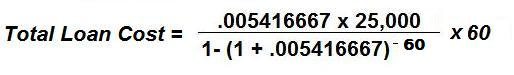CalcuNation

# Total Cost Loan Calculator

## Calculate the total cost over the life of a car loan with this Total Cost Loan Calculator.

Principal Loan Amount:
Yearly Interest Rate: %
Total Months of the Loan:
A 5 year loan has 60 months, a 6 year loan has 72 months.

### How do you find the total cost of an auto loan?

Note: This calculator only shows the total accrued loan cost for the
principal and interest. This does not include other additional costs
associated with insurance or taxes.

How do you find the total loan cost?

To calculate the total loan cost of a vehicle loan use this formula:r = Monthly Interest Rate (in Decimal Form) =
(Yearly Interest Rate/100) / 12

P = Principal Amount on the Loan

N = Total # of Months for the loan ( Years on the loan x 12)

Example: The total cost for 5 year loan, with a principal of \$25,000,
and a yearly interest rate of 6.5%:

r = (6.5 / 100) / 12 = .005416667

P = 25,000

N = (30 x 12) = 60The total cost for the life of the loan is \$29,349.22

### \$100 Promotion

Win \$100 towards teaching supplies! We want to see your websites and blogs.Enter Here

### Calculator Popups

Scientific Calculator
Simple Calculator

### Calculator Ideas

We use your calculator ideas to create new and useful online calculators.
Submit Calculator Idea